Case Based Questions (MCQ)

Chapter 1 Class 10 Real Numbers
Serial order wise

## A garden consists of 135 rose plants planted in certain number of columns. There are another set  of 225 marigold plants, which is to be planted in  the same number of columns.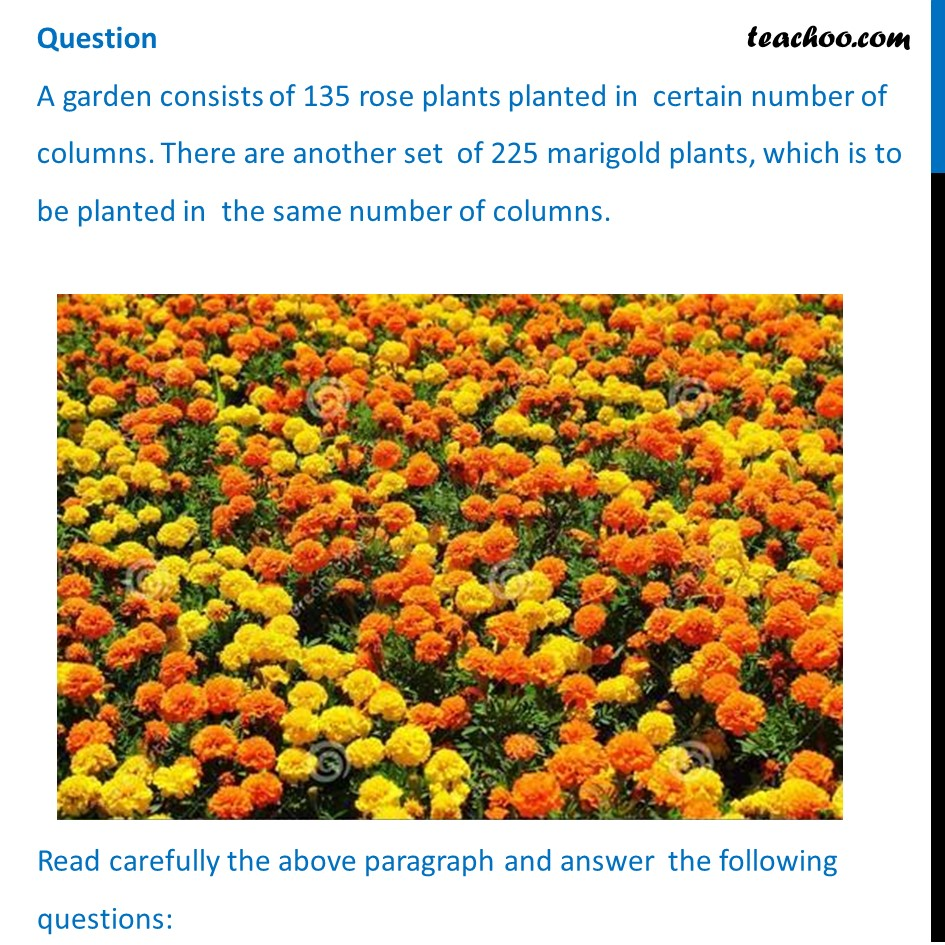## (d) 35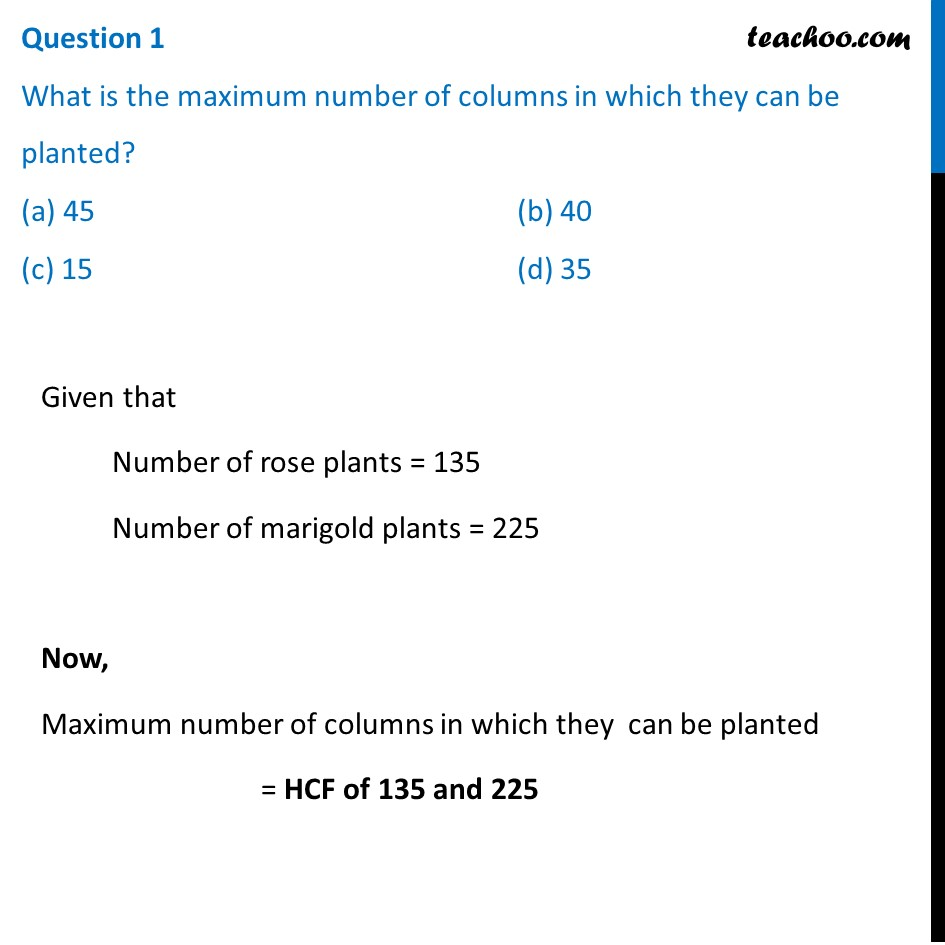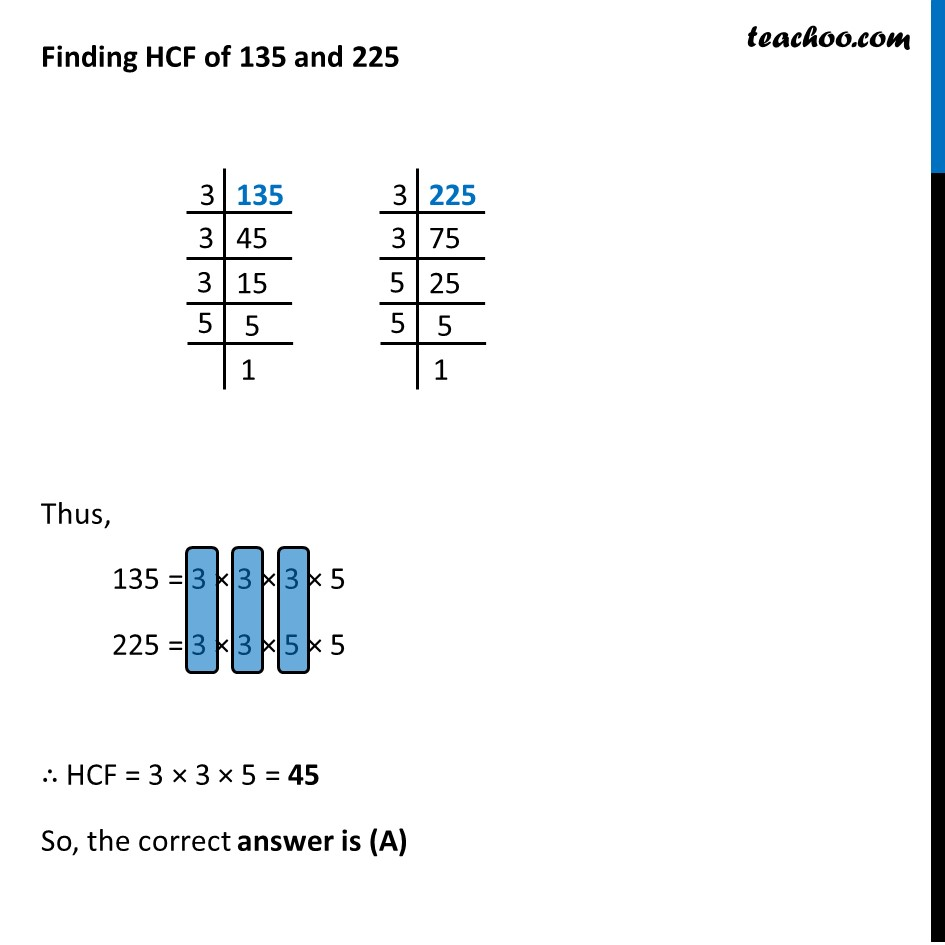## (d) 45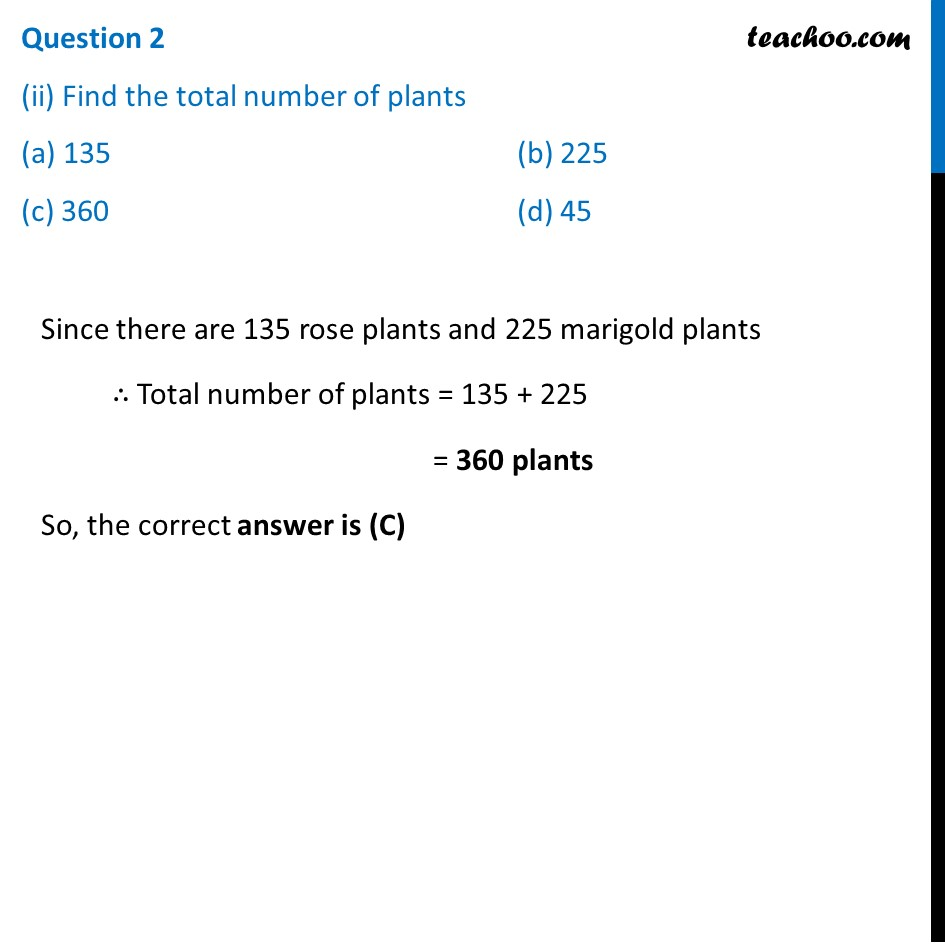## (d) 6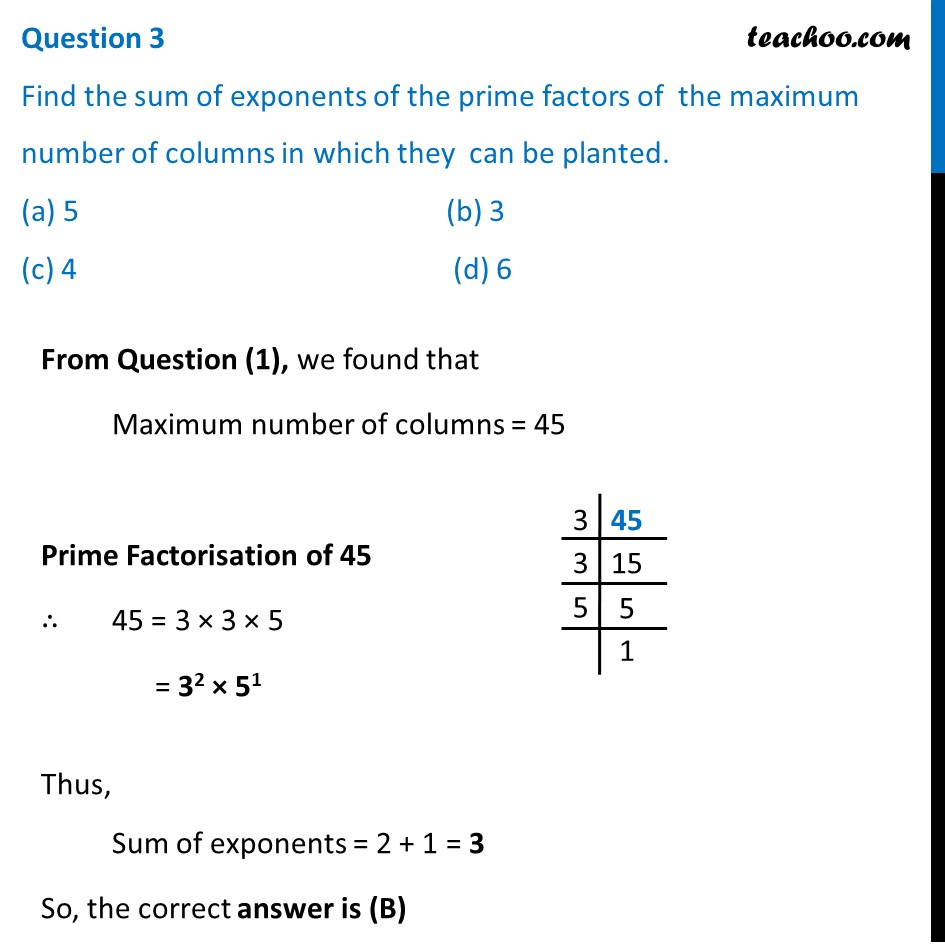##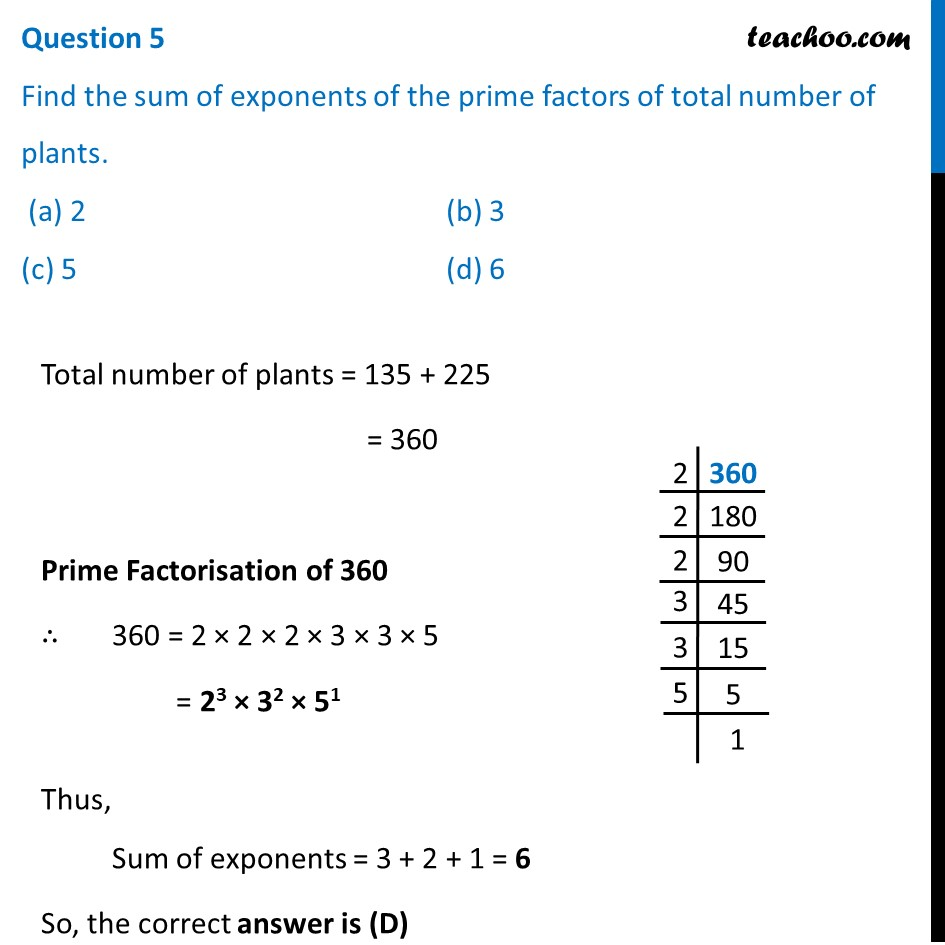Learn in your speed, with individual attention - Teachoo Maths 1-on-1 Class

### Transcript

Question A garden consists of 135 rose plants planted in certain number of columns. There are another set of 225 marigold plants, which is to be planted in the same number of columns. Read carefully the above paragraph and answer the following questions: Question 1 What is the maximum number of columns in which they can be planted? (a) 45 (b) 40 (c) 15 (d) 35 Given that Number of rose plants = 135 Number of marigold plants = 225 Now, Maximum number of columns in which they can be planted = HCF of 135 and 225 Finding HCF of 135 and 225 Thus, 135 = 3 × 3 × 3 × 5 225 = 3 × 3 × 5 × 5 ∴ HCF = 3 × 3 × 5 = 45 So, the correct answer is (A) Question 2 (ii) Find the total number of plants (a) 135 (b) 225 (c) 360 (d) 45 Since there are 135 rose plants and 225 marigold plants ∴ Total number of plants = 135 + 225 = 360 plants So, the correct answer is (C) Question 3 Find the sum of exponents of the prime factors of the maximum number of columns in which they can be planted. (a) 5 (b) 3 (c) 4 (d) 6 From Question (1), we found that Maximum number of columns = 45 Prime Factorisation of 45 ∴ 45 = 3 × 3 × 5 = 32 × 51 Thus, Sum of exponents = 2 + 1 = 3 So, the correct answer is (B) Question 4 What is total numbers of row in which they can be planted (a) 3 (b) 5 (c) 8 (d) 15 From Question 1 Number of plants in 1 row = 45 Rose plants Number of rows of Rose plants = 135/45 = 3 Marigold plants Number of rows of marigold plants = 225/45 = 5 Thus, Total number of rows = 3 + 5 = 8 So, the correct answer is (C) Question 5 Find the sum of exponents of the prime factors of total number of plants. (a) 2 (b) 3 (c) 5 (d) 6 Total number of plants = 135 + 225 = 360 Prime Factorisation of 360 ∴ 360 = 2 × 2 × 2 × 3 × 3 × 5 = 23 × 32 × 51 Thus, Sum of exponents = 3 + 2 + 1 = 6 So, the correct answer is (D)